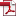## 비트 일부로부터 Multi-Prime RSA와 Prime Power RSA의 개인키를 복구하는 알고리즘

Vol. 26, No. 6, pp. 1401-1412, 12월. 201610.13089/JKIISC.2016.26.6.1401, Full Text:Keywords: Multi-prime RSA, Prime Power RSA, Key Recovery, factorization
Abstract

Multi-Prime RSA와 Prime Power RSA는 변형 RSA 시스템의 일종이며, 이 중에서 Multi-Prime RSA는 서로 다른 r(r>2)개의 소수 $p_1,p_2,{\cdots},p_r$에 대하여 $N=p_1p_2{\cdots}p_r$을, Prime Power RSA는 서로 다른 소수 p, q와 양의 정수 r(>1)에 대하여 $N=p^rq$를 각각 모듈러스로 사용한다. 본 논문에서는 Heninger와 Shacham에 의해 제안된 방법을 사용하여 이 시스템들에 대한 안전성을 분석하며 구체적으로, 만약 $p_1,p_2,{\cdots},p_r$의 전체 비트 중 $2-2^{1/r}$의 비율에 해당하는 비트가 랜덤하게 주어지면 $N=p_1p_2{\cdots}p_r$이 다항식 시간 안에 소인수분해될 수 있음을, 그리고 p, q의 전체 비트 중 $2-{\sqrt{2}}$의 비율에 해당하는 비트가 랜덤하게 주어지면 $N=p^rq$가 다항식 시간 안에 소인수분해될 수 있음을 각각 보인다. 또한 $N=p_1p_2p_3$, $N=p^2q$, $N=p^3q$에 적용한 실험 결과를 통해 본 논문의 결과를 검증한다.

Statistics